# Fraction calculator

The calculator performs basic and advanced operations with fractions, expressions with fractions combined with integers, decimals, and mixed numbers. It also shows detailed step-by-step information about the fraction calculation procedure. Solve problems with two, three, or more fractions and numbers in one expression.

## Result:

### 18/9 - 12/3 = 2/9 ≅ 0.2222222

Spelled result in words is two ninths.

### How do you solve fractions step by step?

1. Conversion a mixed number 1 8/9 to a improper fraction: 1 8/9 = 1 8/9 = 1 · 9 + 8/9 = 9 + 8/9 = 17/9

To find new numerator:
a) Multiply the whole number 1 by the denominator 9. Whole number 1 equally 1 * 9/9 = 9/9
b) Add the answer from previous step 9 to the numerator 8. New numerator is 9 + 8 = 17
c) Write a previous answer (new numerator 17) over the denominator 9.

One and eight ninths is seventeen ninths
2. Conversion a mixed number 1 2/3 to a improper fraction: 1 2/3 = 1 2/3 = 1 · 3 + 2/3 = 3 + 2/3 = 5/3

To find new numerator:
a) Multiply the whole number 1 by the denominator 3. Whole number 1 equally 1 * 3/3 = 3/3
b) Add the answer from previous step 3 to the numerator 2. New numerator is 3 + 2 = 5
c) Write a previous answer (new numerator 5) over the denominator 3.

One and two thirds is five thirds
3. Subtract: 17/9 - 5/3 = 17/9 - 5 · 3/3 · 3 = 17/9 - 15/9 = 17 - 15/9 = 2/9
For adding, subtracting, and comparing fractions, it is suitable to adjust both fractions to a common (equal, identical) denominator. The common denominator you can calculate as the least common multiple of both denominators - LCM(9, 3) = 9. In practice, it is enough to find the common denominator (not necessarily the lowest) by multiplying the denominators: 9 × 3 = 27. In the next intermediate step, the fraction result cannot be further simplified by canceling.
In words - seventeen ninths minus five thirds = two ninths.

#### Rules for expressions with fractions:

Fractions - use the slash “/” between the numerator and denominator, i.e., for five-hundredths, enter 5/100. If you are using mixed numbers, be sure to leave a single space between the whole and fraction part.
The slash separates the numerator (number above a fraction line) and denominator (number below).

Mixed numerals (mixed fractions or mixed numbers) write as non-zero integer separated by one space and fraction i.e., 1 2/3 (having the same sign). An example of a negative mixed fraction: -5 1/2.
Because slash is both signs for fraction line and division, we recommended use colon (:) as the operator of division fractions i.e., 1/2 : 3.

Decimals (decimal numbers) enter with a decimal point . and they are automatically converted to fractions - i.e. 1.45.

The colon : and slash / is the symbol of division. Can be used to divide mixed numbers 1 2/3 : 4 3/8 or can be used for write complex fractions i.e. 1/2 : 1/3.
An asterisk * or × is the symbol for multiplication.
Plus + is addition, minus sign - is subtraction and ()[] is mathematical parentheses.
The exponentiation/power symbol is ^ - for example: (7/8-4/5)^2 = (7/8-4/5)2

#### Examples:

subtracting fractions: 2/3 - 1/2
multiplying fractions: 7/8 * 3/9
dividing Fractions: 1/2 : 3/4
exponentiation of fraction: 3/5^3
fractional exponents: 16 ^ 1/2
adding fractions and mixed numbers: 8/5 + 6 2/7
dividing integer and fraction: 5 ÷ 1/2
complex fractions: 5/8 : 2 2/3
decimal to fraction: 0.625
Fraction to Decimal: 1/4
Fraction to Percent: 1/8 %
comparing fractions: 1/4 2/3
multiplying a fraction by a whole number: 6 * 3/4
square root of a fraction: sqrt(1/16)
reducing or simplifying the fraction (simplification) - dividing the numerator and denominator of a fraction by the same non-zero number - equivalent fraction: 4/22
expression with brackets: 1/3 * (1/2 - 3 3/8)
compound fraction: 3/4 of 5/7
fractions multiple: 2/3 of 3/5
divide to find the quotient: 3/5 ÷ 2/3

The calculator follows well-known rules for order of operations. The most common mnemonics for remembering this order of operations are:
PEMDAS - Parentheses, Exponents, Multiplication, Division, Addition, Subtraction.
BEDMAS - Brackets, Exponents, Division, Multiplication, Addition, Subtraction
BODMAS - Brackets, Of or Order, Division, Multiplication, Addition, Subtraction.
GEMDAS - Grouping Symbols - brackets (){}, Exponents, Multiplication, Division, Addition, Subtraction.
Be careful, always do multiplication and division before addition and subtraction. Some operators (+ and -) and (* and /) has the same priority and then must evaluate from left to right.

## Fractions in word problems:

• EricaErica bought 3 1/2 yards of fabric. If she uses 2/3 of the fabric, how much will she have left?
• Square metal sheetWe cut out four squares of 300 mm side from a square sheet metal plate with a side of 0,7 m. Express the fraction and the percentage of waste from the square metal sheet.
• Animal species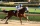Of 100 types of animals, 9/100 were discovered in ancient times, and 2/100 were discovered in the Middle Ages. Another 3/10 were discovered in the 1800s. What fraction of the 100 types of animals was discovered after the 1800s? Explain.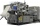Erika’s career consists of filing, typing and answering phones. She spends 1/6 of her time filing and 5/8 of her time typing. What fraction of her time does she spend answering phone calls?
• A laundryMr. Green washed 1/4 of his laundry. His son washed 3/7 of it. Who washed most of the laundry? How much of the laundry still needs to be washed?
• Circular gardenAlice creates a circular vegetable garden. Tomatoes are planted in 1/3 of the circular garden, carrots are planted in 2/5 of the circular garden, and green peppers are planted in 1/10 of the circular garden. What fraction represents the remaining unplante
• Coloured teacups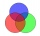The teacups in Tea Stop 55 are `2/5` green and `3/10` yellow. What fraction of the teacups are neither green nor yellow?
• EmployeesOf all 360 employees, there are 11/12 women. How many men work in a company?
• Visit to grandfatherShane's family traveled 3/10 of the distance to his grandfather’s house on Saturday. They traveled 4/7 of the remaining distance on Sunday. What fraction of the total distance to his grandfather’s house was traveled on Sunday?
• Monica’s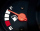Monica's lawnmower had 1/8 of a gallon of gasoline in the tank. Monica started mowing and used all of the gasoline. She put 6/10 of a gallon of gasoline in the tank. After she mowed, 1/4 of a gallon was left in the tank. What was the total amount of gasol
• The BakeryAt a Bakery, ⅗ of the baked goods are pies, and the rest are cakes. ⅓ of the pies are coconut. ⅙ of the cakes are angel food. What fraction of all the baked goods at the Bakery are coconut pies? What fraction of all the baked goods at the Bakery are are a
• Lunch timeIn a cafeteria, 3/10 of the students are eating salads, and 3/5 are eating sandwiches. There are 30 students in the cafeteria. How many students are eating lunches other than salads or sandwiches?
• Sugar 8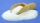Heather has 2 cups of powdered sugar. She sprinkles 3/5 of the sugar onto a plate of brownies and sprinkles the rest into a plate of lemon cookies. How much sugar does Heather sprinkle on the brownies? How much sugar does Heather sprinkle on the lemon coo